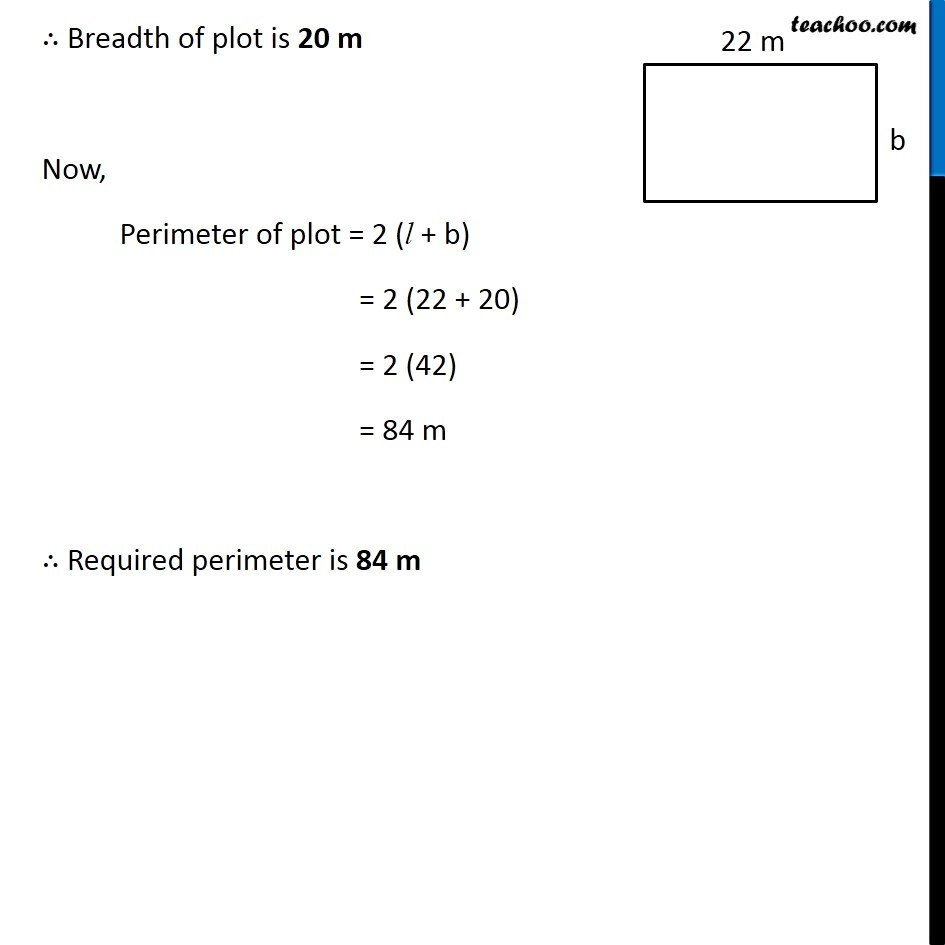Area of Square and Rectangles - Worksheet

Chapter 9 Class 7 Perimeter and Area
Serial order wiseLearn in your speed, with individual attention - Teachoo Maths 1-on-1 Class

### Transcript

Question 3 Find the breadth of a rectangular plot of land, if its area is 440 m2 and the length is 22 m. Also find its perimeter Length of rectangular plot = l = 22 m Breadth of rectangular plot = b = ? Given, Area of plot = 440 m2 l × b = 440 22 × b = 440 b = 440/22 b = 20 ∴ Breadth of plot is 20 m Now, Perimeter of plot = 2 (l + b) = 2 (22 + 20) = 2 (42) = 84 m ∴ Required perimeter is 84 m